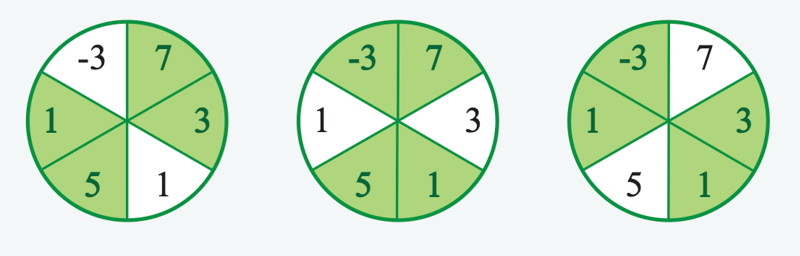# Amazon Online Assessment 2021 (OA) - Max Profit

An Amazon seller is deciding which of their products to invest in for the next quarter to maximize their profits. They have each of their products listed as segments of a circle. Due to varying market conditions, the products do not sell consistently. The seller wants to achieve maximum profit using limited resources for investment. The product list is segmented into a number of equal segments, and a projected profit is calculated for each segment. The projected profit is the cost to invest versus the sale price of the product. The seller has chosen to invest in a number of contiguous segments along with those opposite. Determine the maximum profit the seller can achieve using this approach.For example, the product list Is divided into n = 6 sections and will select k = 2 contiguous sections and those opposite to invest in. The profit estimates are `profit = [1, 5, 1, 3, 7, -3]` respectively. The diagrams below show the possible choices with `profits` at the 9 o'clock position and filling counterclockwise.

The profit levels, from left to right, are `1+5+7+3=16`, `5+1+7+-3=10`, and `1+3+-3+1=2`. The maximum profit is 16.

Relevant Amazon OA Problems:

### Input

• `k`: an integer that denotes half of the needed number of products within the list
• `profit`: an array of integers that denote the profit from investing in each of the products

### Output

the maximum profit possible

### Examples

#### Example 1:

Input:

``````1k = 2
2profit = [1, 5, 1, 3, 7 -3]``````

Output: `16`

Explanation:

The profit levels, from left to right, are `1+5+7+3=16`, `5+1+7+-3=10`, and `1+3+-3+1=2`. The maximum profit is 16.

#### Example 2:

Input:

``````1k = 1
2profit = 3 -5``````

Output: `-2`

Explanation:

Here `k=1` and `n=2`. The seller will choose `2*k=2` products. In this case, it is the entire list, so overall profit is `3+-5=-2`.

### Constraints

• `1 <= k <= n/2`
• `2 <= n <= 10^5`
• n is even
• `0 <= |profit[i]| <= 10^9`

## Title

### Script

Lorem Ipsum is simply dummy text of the printing and typesetting industry. `Lorem` `Ipsum` has been the industry's standard dummy text ever since the 1500s, when an unknown printer took a galley of type and scrambled it to make a type specimen book.

Contrary to popular belief, `Lorem` `Ipsum` is not simply random text.

``````1  >>> a = [1, 2, 3]
2  >>> a[-1]
3  3``````